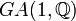General affine group:GA(1,Q)

View a complete list of particular groups (this is a very huge list!)[SHOW MORE]

Definition

This group, denoted$GA(1,\mathbb{Q})$ or$AGL(1,\mathbb{Q})$, is defined in the following equivalent ways:

1. It is the general affine group of degree one over the field of rational numbers$\mathbb{Q}$. Explicitly, it is the group of transformations of the form$x \mapsto ax + b$, where$a \in \mathbb{Q}^*, b \in \mathbb{Q}$, with multiplication defined by composition.
2. It is the holomorph of the (additive) group of rational numbers.
3. It is the external semidirect product$\mathbb{Q} \rtimes \mathbb{Q}^*$ where the latter acts on the former by multiplication.

Group properties

Property Satisfied? Explanation
abelian group No
finitely generated group No
nilpotent group No
metabelian group Yes
solvable group Yes